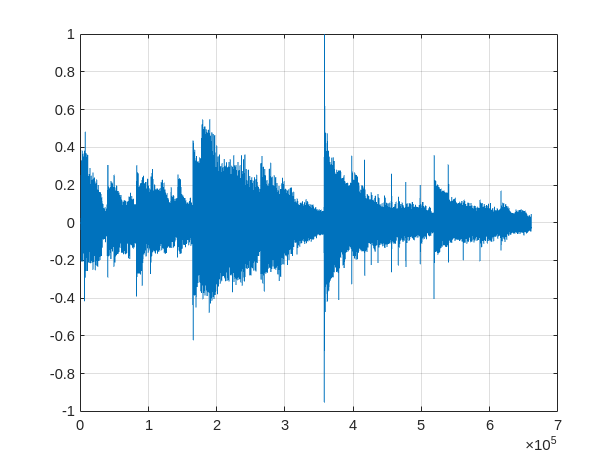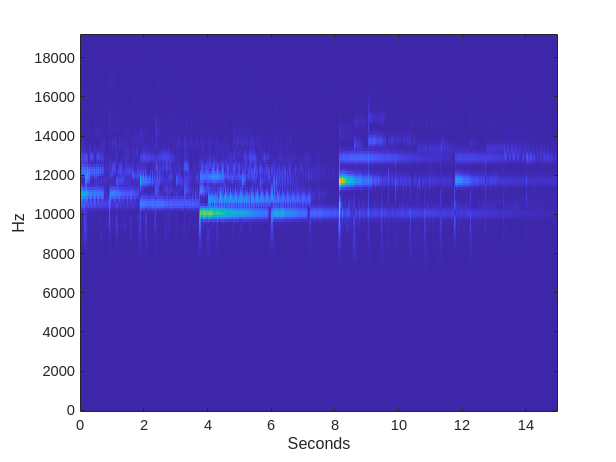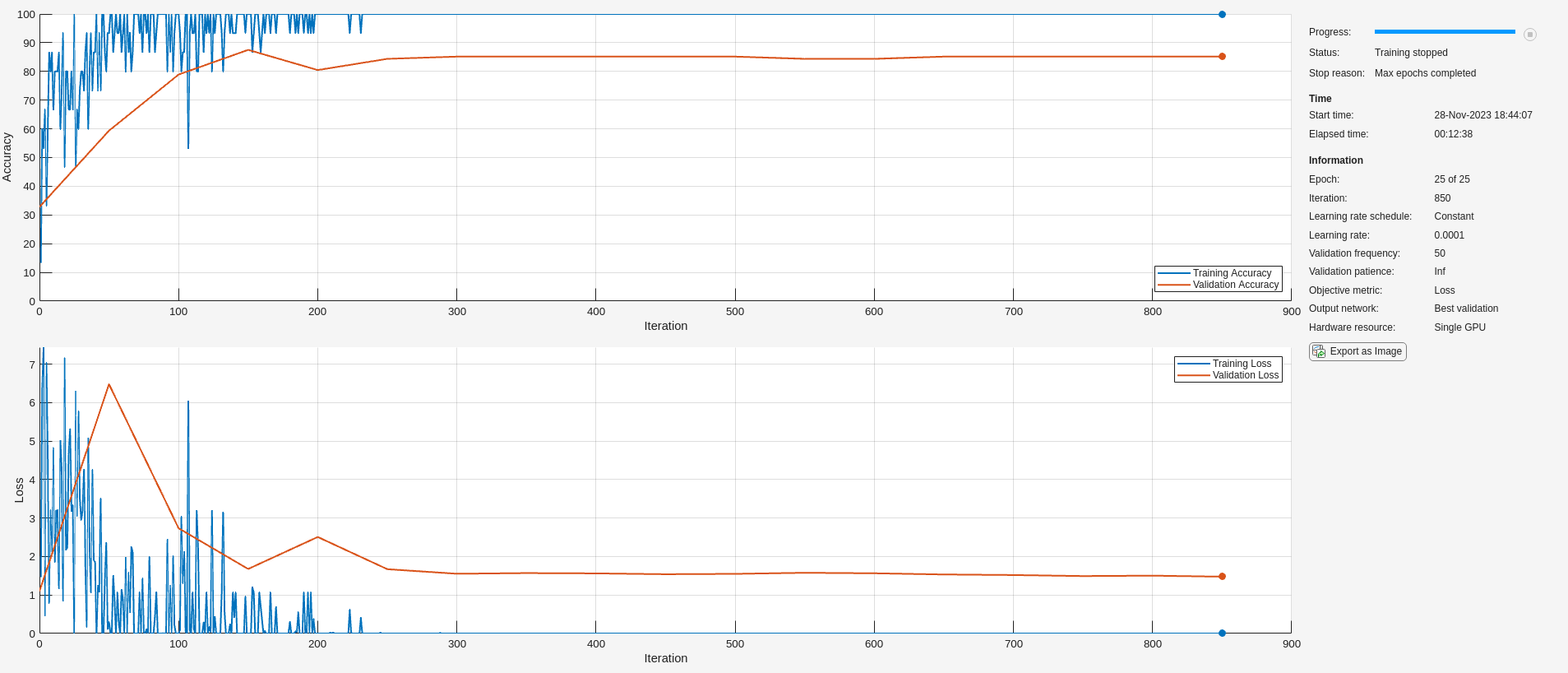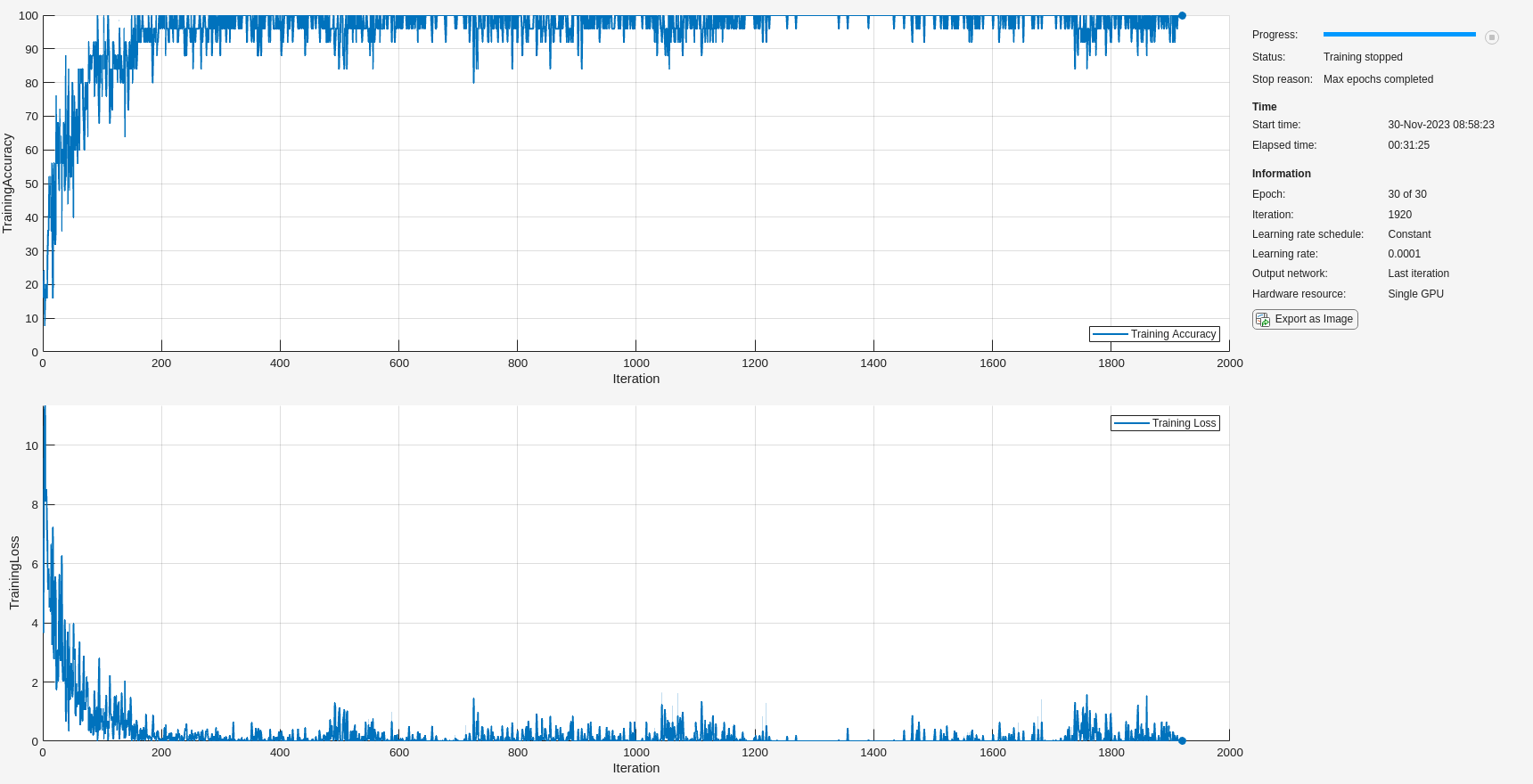# GPU Acceleration of Scalograms for Deep Learning

This example shows how you can accelerate scalogram computation using GPUs. The computed scalograms are used as the input features to deep convolution neural networks (CNN) for ECG and spoken digit classification.

You must have a CUDA-enabled NVIDIA GPU with compute capability 3.0 or higher. See GPU Support by Release (Parallel Computing Toolbox) for details. The audio section of this example requires Audio Toolbox™ to use the audio datastore and transformed datastore.

### Scalogram Computation Using GPU

The most efficient way to compute scalograms on the GPU is to use `cwtfilterbank`. The steps to compute scalograms on the GPU are:

1. Construct `cwtfilterbank` with the desired property settings.

2. Move the signal to the GPU using `gpuArray`.

3. Use the filter bank `WT` method to compute the continuous wavelet transform (CWT).

The first time you use the `WT` method, `cwtfilterbank` caches the wavelet filters on the GPU. As a result, substantial time savings in computation are realized when you obtain scalograms of multiple signals using the same filter bank and same datatype. The following demonstrates the recommended workflow. As an example, use a sample of guitar music containing 661,500 samples.

```[y,fs] = audioread('guitartune.wav'); plot(y) grid on```Because most NVIDIA GPUs are significantly more efficient with single rather than double-precision data, cast the signal to single precision.

`y = single(y);`

Construct the filter bank to match the signal length and the sampling frequency. For deep learning, the sampling frequency is normally not necessary and therefore can be excluded.

`fb = cwtfilterbank('SignalLength',length(y),'SamplingFrequency',fs);`

Finally move the signal to the GPU using `gpuArray `and compute the CWT of the data. Plot the resulting scalogram.

```[cfs,f] = fb.wt(gpuArray(y)); t = 0:1/fs:(length(y)*1/fs)-1/fs; imagesc(t,f,abs(cfs)) axis xy ylabel('Hz') xlabel('Seconds')```Use `gather` to bring the CWT coefficients and any other outputs back to the CPU.

```cfs = gather(cfs); f = gather(f);```

To demonstrate the efficiency gained in using the GPU, time the CWT computation on the GPU and CPU for the same signal. The GPU compute times reported here are obtained using an NVIDIA Titan V with a compute capability of 7.0.

```ygpu = gpuArray(y); fgpu = @()fb.wt(ygpu); Tgpu = gputimeit(fgpu)```
```Tgpu = 0.2658 ```

Repeat the same measurement on the CPU and examine the ratio of GPU to CPU time to see the reduction in computation time.

```fcpu = @()fb.wt(y); Tcpu = timeit(fcpu)```
```Tcpu = 3.7088 ```
`Tcpu/Tgpu`
```ans = 13.9533 ```

### Scalograms in Deep Learning

A common application of the CWT in deep learning is to use the scalogram of a signal as the input "image" to a deep CNN. This necessarily mandates the computation of multiple scalograms, one for each signal in the training, validation, and test sets. While GPUs are often used to speed up training and inference in the deep network, it is also beneficial to use GPUs to accelerate any feature extraction, or data preprocessing needed to make the deep network more robust.

To illustrate this, the following section applies wavelet scalograms to human electrocardiogram (ECG) classification. Scalograms are used with the same data treated in Classify Time Series Using Wavelet Analysis and Deep Learning. In that example, transfer learning with GoogLeNet and SqueezeNet was used to classify ECG waveforms into one of three categories. The description of the data and how to obtain it is repeated here for convenience.

The ECG data is obtained from three groups of people: persons with cardiac arrhythmia (ARR), persons with congestive heart failure (CHF), and persons with normal sinus rhythms (NSR). In total there are 162 ECG recordings from three PhysioNet databases: MIT-BIH Arrhythmia Database , MIT-BIH Normal Sinus Rhythm Database , and The BIDMC Congestive Heart Failure Database . More specifically, 96 recordings from persons with arrhythmia, 30 recordings from persons with congestive heart failure, and 36 recordings from persons with normal sinus rhythms. The goal is to train a model to distinguish between ARR, CHF, and NSR.

You can obtain this data from the MathWorks GitHub repository. To download the data from the website, click `Clone or download` and select `Download ZIP`. Save the file `physionet_ECG_data-master.zip` in a folder where you have write permission. The instructions for this example assume you have downloaded the file to your temporary directory, `tempdir`, in MATLAB. Modify the subsequent instructions for unzipping and loading the data if you choose to download the data in folder different from `tempdir`. If you are familiar with Git, you can download the latest version of the tools (git) and obtain the data from a system command prompt using `git clone`` ``https://github.com/mathworks/physionet_ECG_data/`.

`unzip(fullfile(tempdir,'physionet_ECG_data-master.zip'),tempdir)`

Unzipping creates the folder `physionet-ECG_data-master` in your temporary directory. This folder contains the text file `README.md` and `ECGData.zip`. The `ECGData.zip` file contains

• `ECGData.mat`

• `Modified_physionet_data.txt`

• `License.txt`

`ECGData.mat` holds the data used in this example. The text file, `Modified_physionet_data.txt`, is required by PhysioNet's copying policy and provides the source attributions for the data as well as a description of the preprocessing steps applied to each ECG recording.

Unzip `ECGData.zip` in `physionet-ECG_data-master`. Load the data file into your MATLAB workspace.

```unzip(fullfile(tempdir,'physionet_ECG_data-master','ECGData.zip'),... fullfile(tempdir,'physionet_ECG_data-master')) load(fullfile(tempdir,'physionet_ECG_data-master','ECGData.mat'))```

`ECGData` is a structure array with two fields: `Data` and `Labels`. The `Data` field is a 162-by-65536 matrix where each row is an ECG recording sampled at 128 hertz. `Labels` is a 162-by-1 cell array of diagnostic labels, one for each row of `Data`. The three diagnostic categories are: `'ARR'`, `'CHF'`, and `'NSR'`. Use the helper function, `helperRandomSplit`, to split the data into training and validation sets with 80% of the data allocated for training and 20% for validation. Convert the ECG diagnostic labels into categoricals.

```[trainData, validationData, trainLabels, validationLabels] = helperRandomSplit(80,ECGData); trainLabels = categorical(trainLabels); validationLabels = categorical(validationLabels);```

There are 130 records in the `trainData` set and 32 records in validation`Data`. By design, the training data contains 80.25% (130/162) of the data. Recall that the ARR class represents 59.26% of the data (96/162), the CHF class represents 18.52% (30/162), and the NSR class represents 22.22% (36/162). Examine the percentage of each class in the training and test sets. The percentages in each are consistent with the overall class percentages in the data set.

`Ctrain = countcats(trainLabels)./numel(trainLabels).*100`
```Ctrain = 3×1 59.2308 18.4615 22.3077 ```
`Cvalid = countcats(validationLabels)./numel(validationLabels).*100`
```Cvalid = 3×1 59.3750 18.7500 21.8750 ```

### Scalograms With Deep CNN — ECG Data

#### Scalogram Computation on the GPU

Compute the scalograms for both the training and validation sets. Set `useGPU `to `true` to use the GPU and `false `to compute the scalograms on the CPU. To mitigate the effect of large input matrices on the CNN and create more training and validation examples, `helperECGScalograms `splits each ECG waveform into four nonoverlapping segments of 16384 samples each and computes scalograms for all four segments. Replicate the labels to match the expanded dataset. In this case, obtain an estimate of the expended computation time.

```frameLength = 16384; useGPU = true; tic; Xtrain = helperECGScalograms(trainData,frameLength,useGPU);```
```Computing scalograms... Processed 50 files out of 130 Processed 100 files out of 130 ...done ```
```T = toc; sprintf('Elapsed time is %1.2f seconds',T)```
```ans = 'Elapsed time is 4.22 seconds' ```
`trainLabels = repelem(trainLabels,4);`

With the Titan V GPU, 502 scalograms have been computed in approximately 4.2 seconds. Setting `useGPU `to `false `and repeating the above computation demonstrates the speed up obtained by using the GPU. In this case, using the CPU required 33.3 seconds to compute the scalograms. The GPU computation was more than 7 times faster.

Repeat the same process for the validation data.

```useGPU = true; Xvalid = helperECGScalograms(validationData,frameLength,useGPU);```
```Computing scalograms... ...done ```
`validationLabels = repelem(validationLabels,4);`

Next set up a deep CNN to process both the training and validation sets. The simple network used here is not optimized. This CNN is only used to illustrate the end-to-end workflow for cases where the scalograms fit in memory.

```sz = size(Xtrain); specSize = sz(1:2); imageSize = [specSize 1]; dropoutProb = 0.3; layers = [ imageInputLayer(imageSize) convolution2dLayer(3,12,'Padding','same') batchNormalizationLayer reluLayer maxPooling2dLayer(2,'Stride',2) convolution2dLayer(3,20,'Padding','same') batchNormalizationLayer reluLayer maxPooling2dLayer(2,'Stride',2) convolution2dLayer(3,32,'Padding','same') batchNormalizationLayer reluLayer dropoutLayer(dropoutProb) fullyConnectedLayer(3) softmaxLayer classificationLayer];```

Use the following training options.

```options = trainingOptions('sgdm',... 'InitialLearnRate', 1e-4,... 'LearnRateDropPeriod',18,... 'MiniBatchSize', 20,... 'MaxEpochs',25,... 'L2Regularization',1e-1,... 'Plots', 'training-progress',... 'Verbose',false,... 'Shuffle','every-epoch',... 'ExecutionEnvironment','auto',... 'ValidationData',{Xvalid,validationLabels});```

Train the network and measure the validation error.

`trainNetwork(Xtrain,trainLabels,layers,options);`Even though the simple CNN used here is not optimized, the validation accuracy is consistently in the high 80 to low 90 percent range. This is comparable to the validation accuracy achieved with the more powerful and optimized SqueezeNet shown in Classify Time Series Using Wavelet Analysis and Deep Learning example. Further, this is a much more efficient use of the scalogram, because in that example the scalograms had to be rescaled as RGB images compatible with SqueezeNet, saved to disk in an appropriate image format, and then fed to the deep network using `imageDatastore`.

### Spoken Digit Recognition — GPU Computing using Transform Datastore

This section shows how to accelerate scalogram computation using GPU in a transformed datastore workflow.

#### Data

Clone or download the Free Spoken Digit Dataset (FSDD), available at https://github.com/Jakobovski/free-spoken-digit-dataset. FSDD is an open data set, which means that it can grow over time. This example uses the version committed on 01/29/2019 which consists of 2000 recordings of the English digits 0 through 9 obtained from four speakers. Two of the speakers in this version are native speakers of American English and two speakers are nonnative speakers of English with a Belgium French and German accent. The data is sampled at 8000 Hz.

For other approaches to this dataset including wavelet scattering, see Spoken Digit Recognition with Wavelet Scattering and Deep Learning.

Use `audioDatastore` to manage data access and ensure the random division of the recordings into training and test sets. Set the `location` property to the location of the FSDD recordings folder on your computer, for example:

```pathToRecordingsFolder = '/home/user/free-spoken-digit-dataset/recordings'; location = pathToRecordingsFolder;```

Point `audioDatastore `to that location.

`ads = audioDatastore(location);`

The helper function `helpergenLabels `creates a categorical array of labels from the FSDD files. List the classes and the number of examples in each class.

```ads.Labels = helpergenLabels(ads); summary(ads.Labels)```
``` 0 200 1 200 2 200 3 200 4 200 5 200 6 200 7 200 8 200 9 200 ```

#### Transformed Datastore

First split the FSDD into training and test sets. Allocate 80% of the data to the training set and retain 20% for the test set.

```rng default; ads = shuffle(ads); [adsTrain,adsTest] = splitEachLabel(ads,0.8); countEachLabel(adsTrain)```
```ans=10×2 table Label Count _____ _____ 0 160 1 160 2 160 3 160 4 160 5 160 6 160 7 160 8 160 9 160 ```

Next create the CWT filter bank and the transformed datastores for both the training and test data using the helper function, `helperDigitScalogram`. The transformed datastore converts each recording into a signal of length 8192, computes the scalogram on the GPU, and gathers the data back onto the CPU.

```reset(gpuDevice(1)) fb = cwtfilterbank('SignalLength',8192); adsSCTrain = transform(adsTrain,@(audio,info)helperDigitScalogram(audio,info,fb),'IncludeInfo',true); adsSCTest = transform(adsTest,@(audio,info)helperDigitScalogram(audio,info,fb),'IncludeInfo',true);```

#### Deep CNN

Construct a deep CNN to train with the transformed datastore, `adscTrain`. As in the first example, the network is not optimized. The point is to show the workflow using scalograms computed on the GPU for out-of-memory data.

```numClasses = 10; dropoutProb = 0.2; numF = 12; layers = [ imageInputLayer([101 8192 1]) convolution2dLayer(5,numF,'Padding','same') batchNormalizationLayer reluLayer maxPooling2dLayer(3,'Stride',2,'Padding','same') convolution2dLayer(3,2*numF,'Padding','same') batchNormalizationLayer reluLayer maxPooling2dLayer(3,'Stride',2,'Padding','same') convolution2dLayer(3,4*numF,'Padding','same') batchNormalizationLayer reluLayer maxPooling2dLayer(3,'Stride',2,'Padding','same') convolution2dLayer(3,4*numF,'Padding','same') batchNormalizationLayer reluLayer convolution2dLayer(3,4*numF,'Padding','same') batchNormalizationLayer reluLayer maxPooling2dLayer(2) dropoutLayer(dropoutProb) fullyConnectedLayer(numClasses) softmaxLayer classificationLayer('Classes',categories(ads.Labels)); ]; ```

Set the training options for the network.

```miniBatchSize = 25; options = trainingOptions('adam', ... 'InitialLearnRate',1e-4, ... 'MaxEpochs',30, ... 'MiniBatchSize',miniBatchSize, ... 'Shuffle','every-epoch', ... 'Plots', 'training-progress',... 'Verbose',false,... 'ExecutionEnvironment','gpu');```

Train the network.

`trainedNet = trainNetwork(adsSCTrain,layers,options);`In this instance, training was completed in 25 minutes and 10 seconds. If you comment out the call to `gpuArray `in` helperDigitScalogram `and use the CPU to obtain the scalograms, training time increases significantly. In this case, an increase from 25 minutes and 10 seconds to 45 minutes and 38 seconds was observed.

Use the trained network to predict the digit labels for the test set.

```Ypredicted = classify(trainedNet,adsSCTest,'ExecutionEnvironment','CPU'); cnnAccuracy = sum(Ypredicted == adsTest.Labels)/numel(Ypredicted)*100```
```cnnAccuracy = 96.2500 ```

The inference time using the GPU was approximately 22 seconds. Using the CPU, inference time doubled to 45 seconds.

The performance of trained network on the test data is close to 96%. This is comparable to the performance in Spoken Digit Recognition with Wavelet Scattering and Deep Learning.

### Summary

This example has showcased how to use the GPU to accelerate scalogram computation. The example presented the optimal workflow for efficiently computing scalograms both for in-memory data and for out-of-memory data read from disk using transformed datastores.

### References

1. Baim, D. S., W. S. Colucci, E. S. Monrad, H. S. Smith, R. F. Wright, A. Lanoue, D. F. Gauthier, B. J. Ransil, W. Grossman, and E. Braunwald. "Survival of patients with severe congestive heart failure treated with oral milrinone." Journal of the American College of Cardiology. Vol. 7, Number 3, 1986, pp. 661–670.

2. Goldberger A. L., L. A. N. Amaral, L. Glass, J. M. Hausdorff, P. Ch. Ivanov, R. G. Mark, J. E. Mietus, G. B. Moody, C.-K. Peng, and H. E. Stanley. "PhysioBank, PhysioToolkit,and PhysioNet: Components of a New Research Resource for Complex Physiologic Signals." Circulation. Vol. 101, Number 23: e215–e220. [Circulation Electronic Pages; `http://circ.ahajournals.org/content/101/23/e215.full`]; 2000 (June 13). doi: 10.1161/01.CIR.101.23.e215.

3. Moody, G. B., and R. G. Mark. "The impact of the MIT-BIH Arrhythmia Database." IEEE Engineering in Medicine and Biology Magazine. Vol. 20. Number 3, May-June 2001, pp. 45–50. (PMID: 11446209)

```function Labels = helpergenLabels(ads) % This function is only for use in Wavelet Toolbox examples. It may be % changed or removed in a future release. tmp = cell(numel(ads.Files),1); expression = "[0-9]+_"; for nf = 1:numel(ads.Files) idx = regexp(ads.Files{nf},expression); tmp{nf} = ads.Files{nf}(idx); end Labels = categorical(tmp); end```
```function X = helperECGScalograms(data,window,useGPU) disp("Computing scalograms..."); Nsig = size(data,1); Nsamp = size(data,2); Nsegment = Nsamp/window; fb = cwtfilterbank('SignalLength',window,'Voices',10); Ns = length(fb.Scales); X = zeros([Ns,window,1,Nsig*Nsegment],'single'); start = 0; if useGPU data = gpuArray(single(data')); else data = single(data'); end for ii = 1:Nsig ts = data(:,ii); ts = reshape(ts,window,Nsegment); ts = (ts-mean(ts))./max(abs(ts)); for kk = 1:size(ts,2) cfs = fb.wt(ts(:,kk)); X(:,:,1,kk+start) = gather(abs(cfs)); end start = start+Nsegment; if mod(ii,50) == 0 disp("Processed " + ii + " files out of " + Nsig) end end disp("...done"); data = gather(data); end```
```function [x,info] = helperReadSPData(x,info) % This function is only for use Wavelet Toolbox examples. It may change or % be removed in a future release. N = numel(x); if N > 8192 x = x(1:8192); elseif N < 8192 pad = 8192-N; prepad = floor(pad/2); postpad = ceil(pad/2); x = [zeros(prepad,1) ; x ; zeros(postpad,1)]; end x = x./max(abs(x)); end```
```function [dataout,info] = helperDigitScalogram(audioin,info,fb) audioin = single(audioin); audioin = gpuArray(audioin); audioin = helperReadSPData(audioin); cfs = gather(abs(fb.wt(audioin))); audioin = gather(audioin); dataout = {cfs,info.Label}; end```
﻿
##### Support